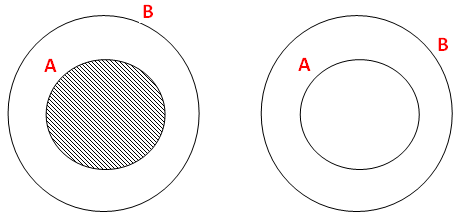### If A ⊂ B, then find A ∩ B and A \ B (use venn diagram)

Question 2 :
If A ⊂ B, then find A ∩ B and A \ B (use venn diagram)
Solution :
Since A is the subset of B we have to draw a small circle A inside the large circle B.
A ∩ B means we have to shade common part of A and B. From this we will get
A ∩ B  = A